# How to Calculate and Solve for Total Storage | Hydrology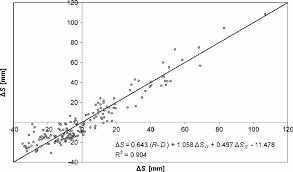The image above represents total storage.

To compute for total storage, three essential parameters are needed and these paramters are Storage co-efficient (k), Dimensionless constant (x) and Average outflow rate (O).

The formula for calculating total storage:

s = k[x + (1 – x)O]

Where:

s = Total Storage
k = Storage Co-efficient
x = Dimensionless Constant
O = Average Outflow Rate

Let’s solve an example;
Find the total storage when the storage co-efficient is 12, the dimensionless constant is 10 and the average outflow rate is 6.

This implies that;

k = Storage Co-efficient = 12
x = Dimensionless Constant = 10
O = Average Outflow Rate = 6

s = k[x + (1 – x)O]
s = 12[10 + (1 – 10)6]
s = 12[10 + (-9)6]
s = 12[10 + -54]
s = 12[-44]
s = -528

Therefore, the total storage is -528.

Calculating the Storage Co-efficient when the Total Storage, the Dimensionless Constant and the Average Outflow Rate is Given.

k = s / [x + (1 – x)O]

Where;

k = Storage Co-efficient
s = Total Storage
x = Dimensionless Constant
O = Average Outflow Rate

Let’s solve an example;
Find the storage co-efficient when the total storage is 32, the dimensionless constant is 8 and the average outflow rate is 4.

This implies that;

s = Total Storage = 32
x = Dimensionless Constant = 8
O = Average Outflow Rate = 4

k = s / [x + (1 – x)O]
k = 32 / [8 + (1 – 8)4]
k = 32 / [8 + (-7)4]
k = 32 / [8 + -28]
k = 32 / [-20]
k = -1.6

Therefore, the storage co-efficient is -1.6.

Nickzom Calculator – The Calculator Encyclopedia is capable of calculating the total storage.

To get the answer and workings of the total storage using the Nickzom Calculator – The Calculator Encyclopedia. First, you need to obtain the app.

You can get this app via any of these means:

To get access to the professional version via web, you need to register and subscribe for NGN 2,000 per annum to have utter access to all functionalities.
You can also try the demo version via https://www.nickzom.org/calculator

Apple (Paid) – https://itunes.apple.com/us/app/nickzom-calculator/id1331162702?mt=8
Once, you have obtained the calculator encyclopedia app, proceed to the Calculator Map, then click on Agricultural under Engineering.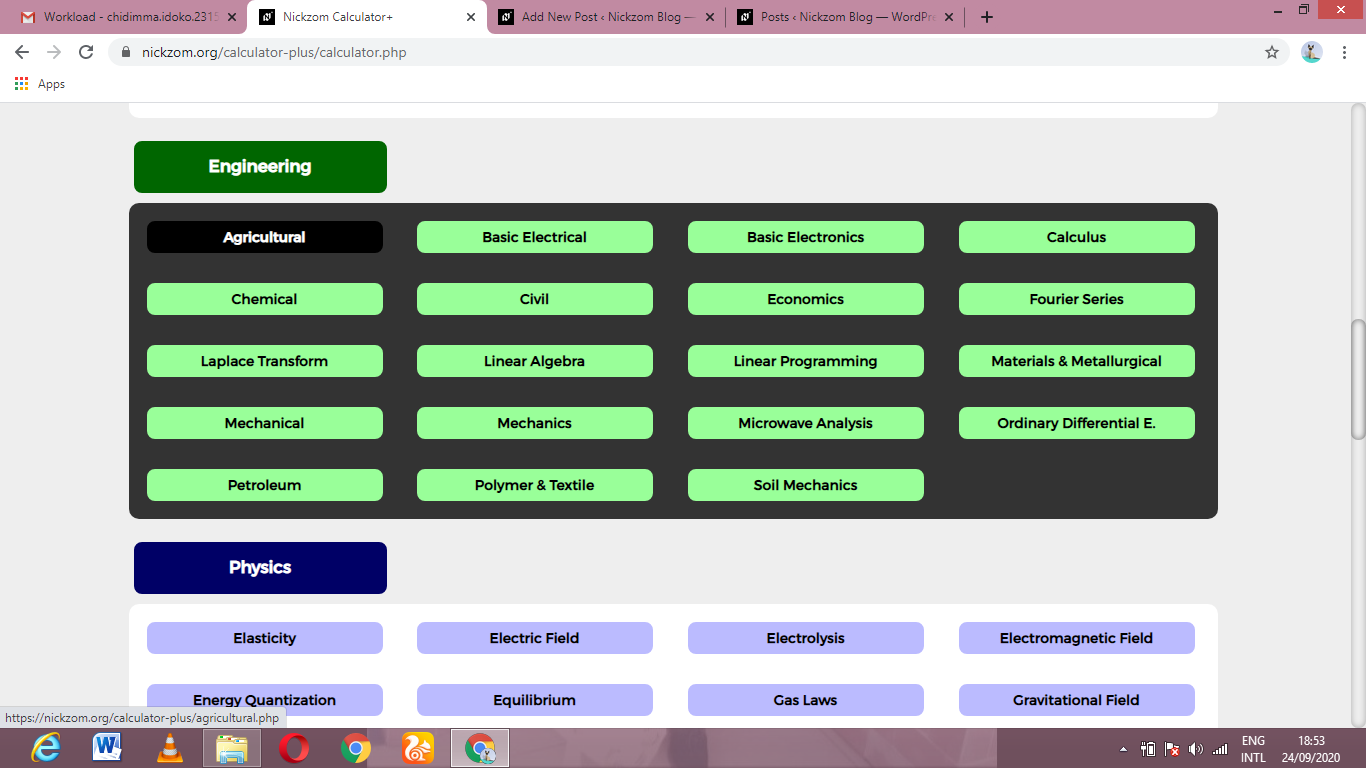Now, Click on Hydrology II under Agricultural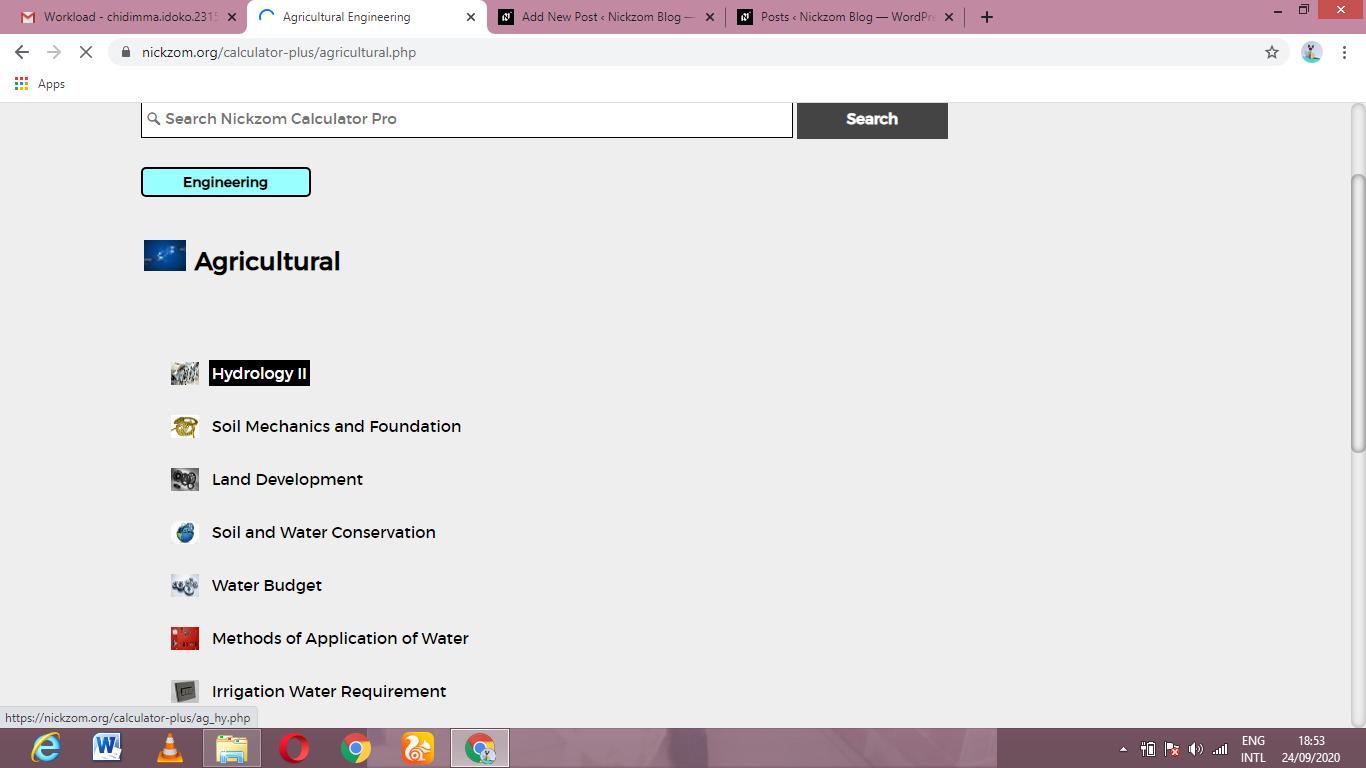Now, Click on Total Storage under Hydrology IIThe screenshot below displays the page or activity to enter your values, to get the answer for the total storage according to the respective parameters which is the Storage co-efficient (k), Dimensionless constant (x) and Average outflow rate (O).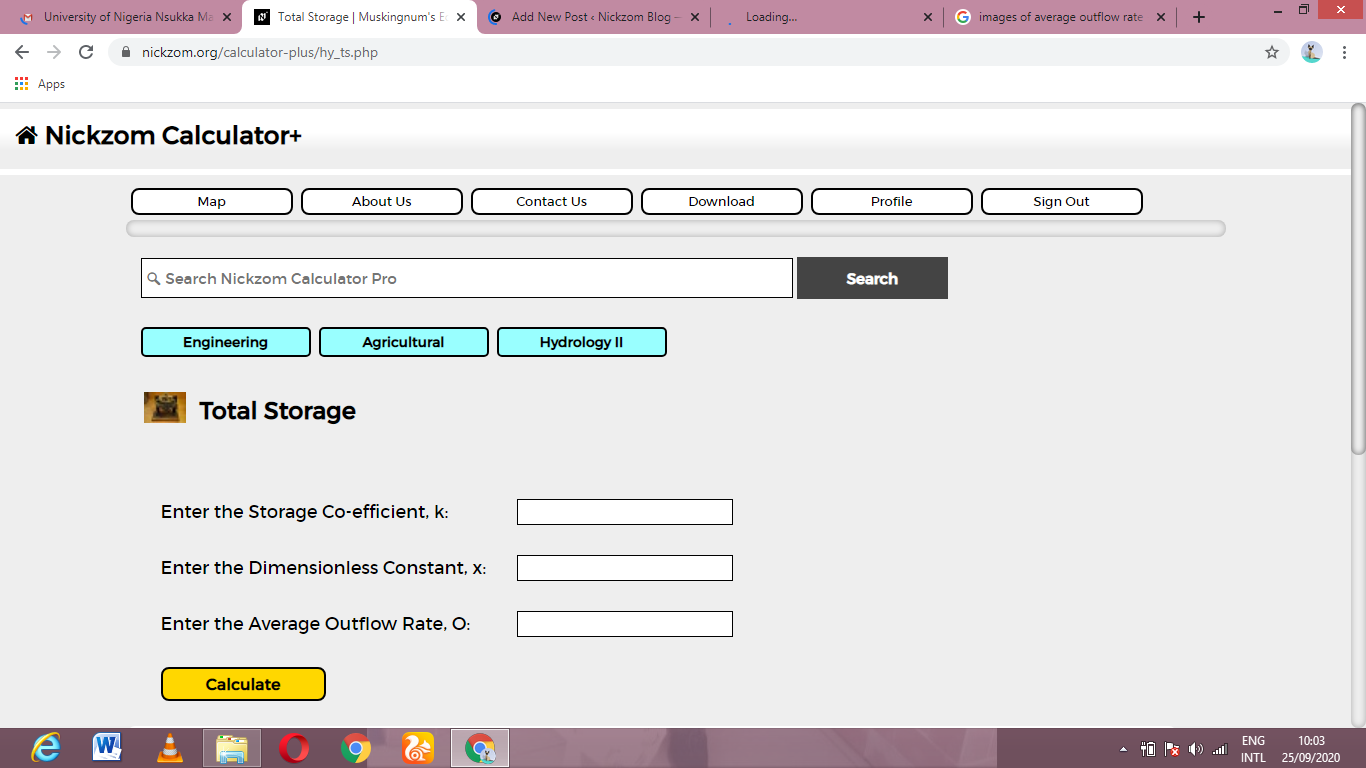Now, enter the values appropriately and accordingly for the parameters as required by the Storage co-efficient (k) is 12, Dimensionless constant (x) is 10 and Average outflow rate (O) is 6.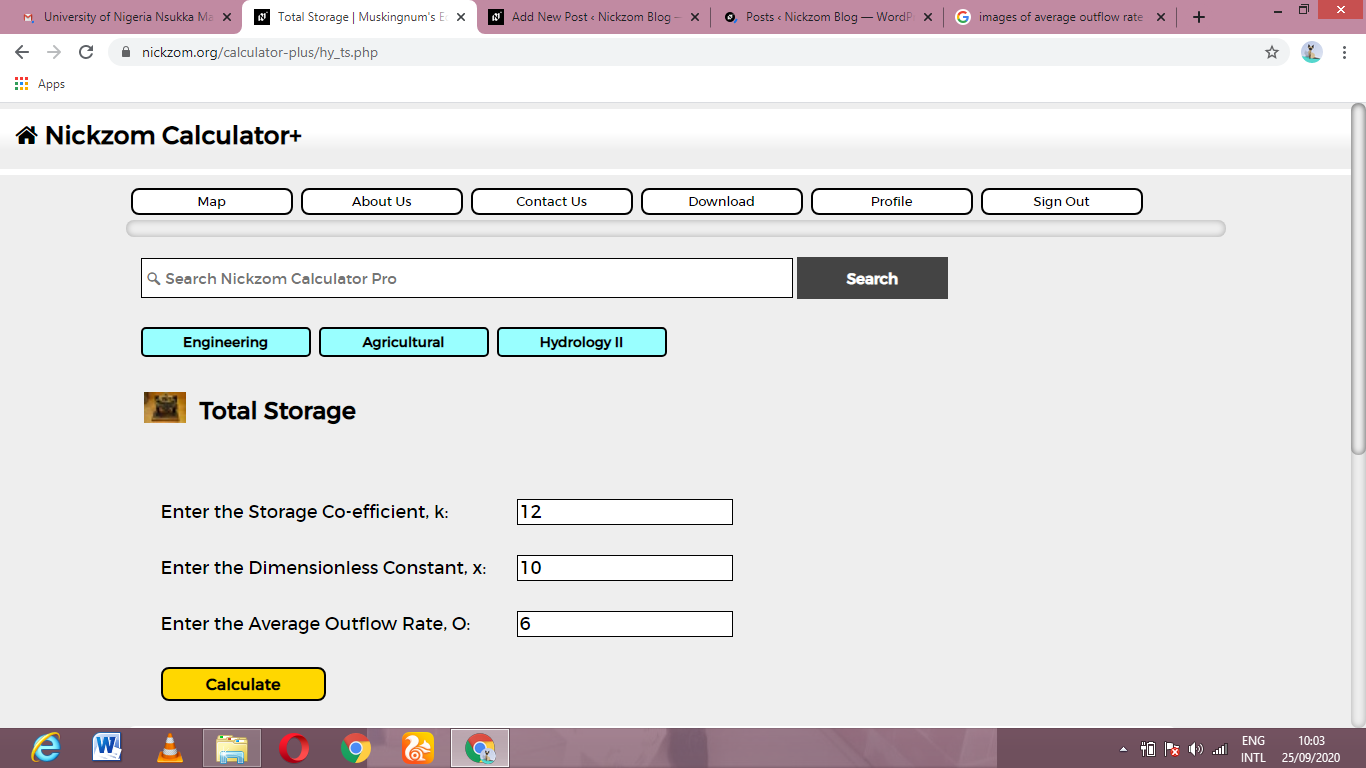Finally, Click on Calculate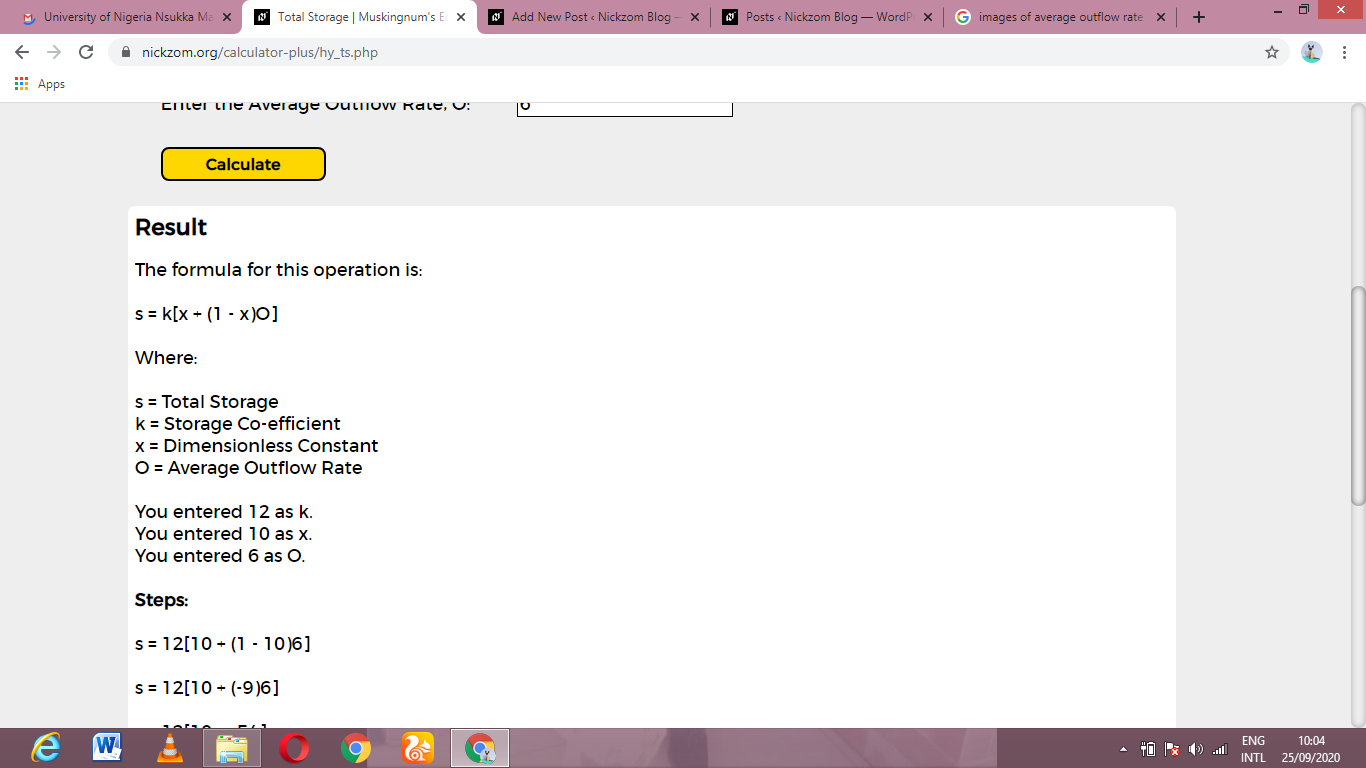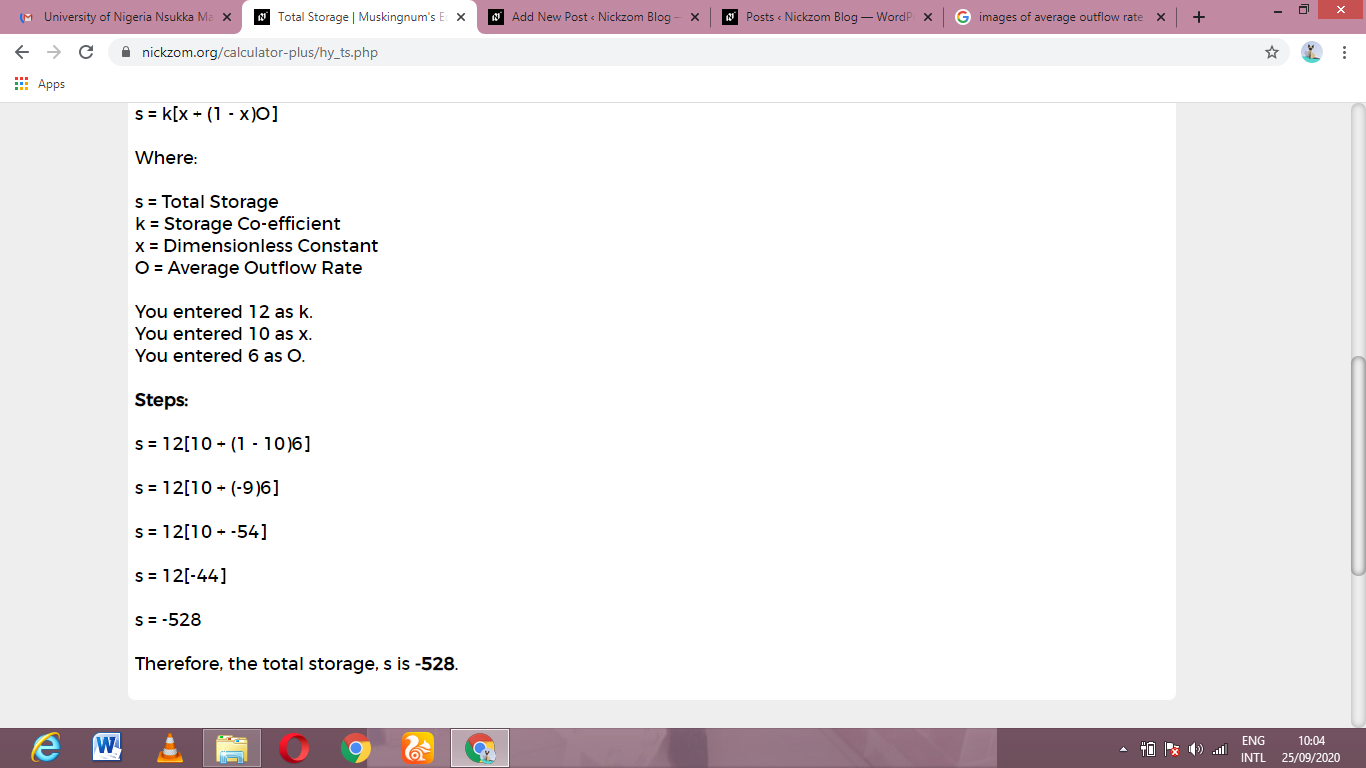As you can see from the screenshot above, Nickzom Calculator– The Calculator Encyclopedia solves for the total storage and presents the formula, workings and steps too.